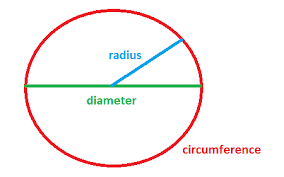# Haskell Program to Find the Perimeter of a Circle

This tutorial discusses writing a program to find the perimeter of a circle in the Haskell programming language.

The perimeter of the circle is the length of the boundary of the circle. The perimeter of a circle is also called the circumference. The perimeter of a circle is defined as 2*pi*r where r is the radius of the circle. For example area of the circle with a radius of 4 units is 25.13274 (2*pi*4).In this tutorial, we see two ways to implement a program to find the perimeter of a circle.

• Program to find the perimeter of a circle using the radius.
• Program to find the perimeter of a circle using the diameter.

## Algorithm Steps

• Take input or initialize the variables.
• Implement the program logic to compute the perimeter of the circle.
• Print or display the perimeter.

## Example 1

Program to find the perimeter of a circle using the radius

main :: IO()
main = do
-- declaring and initializing variables for radius
-- computing the area
print ("The perimeter of the circle with radius "++ show radius ++ " is:")
print (perimeter)


## Output

"The perimeter of the circle with radius 4.0 is:"
25.132741228718345


In the above program, we declared and initialized a variable for the radius of the circle as the radius with the value of 4. We computed the perimeter of the circle using the appropriate logic and loaded the computed perimeter into a variable perimeter. Finally, printed the computed perimeter using the print function. The function print takes the argument as a string and displays it on the display console.

Note − The function show takes the argument of a number and returns the parsed string of a number. “++” is an operator to concatenate strings in Haskell.

## Example 2

Program to find the perimeter of a circle using the diameter

main :: IO()
main = do
-- declaring and initializing variables for radius
let diameter = 4
-- computing the area
let perimeter = pi*diameter
print ("The perimeter of the circle with diameter "++ show diameter ++ " is:")
print (perimeter)


## Output

"The perimeter of the circle with diameter 4.0 is:"
12.566370614359172


As the diameter is defined as 2*radius. The perimeter of a circle can be computed by pi*d, where d is the diameter of a circle.

In the above program, we declared and initialized the variable for the diameter of the circle as a diameter with the value of 4. We computed the perimeter of the circle using the appropriate logic and loaded the computed perimeter into a variable perimeter. Finally, printed the computed perimeter using the print function. The function print takes the argument as a string and displays it on the display console.

## Conclusion

In this tutorial, we discussed two ways to implement a program to find or compute the perimeter of a circle in the Haskell Programming Language.